# pylops.optimization.leastsquares.regularized_inversion#

pylops.optimization.leastsquares.regularized_inversion(Op, y, Regs, x0=None, Weight=None, dataregs=None, epsRs=None, engine='scipy', show=False, **kwargs_solver)[source]#

Regularized inversion.

Solve a system of regularized equations given the operator Op, a data weighting operator Weight, and a list of regularization terms Regs.

Parameters
Oppylops.LinearOperator

Operator to invert of size $$[N \times M]$$

ynumpy.ndarray

Data of size $$[N \times 1]$$

Regslist

Regularization operators (None to avoid adding regularization)

x0numpy.ndarray, optional

Initial guess of size $$[M \times 1]$$

Weightpylops.LinearOperator, optional

Weight operator

dataregslist, optional

Regularization data (if None a zero data will be used for every regularization operator in Regs)

epsRslist, optional

Regularization dampings

enginestr, optional

Solver to use (scipy or pylops)

showbool, optional

Display normal equations solver log

**kwargs_solver

Arbitrary keyword arguments for chosen solver (scipy.sparse.linalg.lsqr and pylops.optimization.solver.cgls are used for engine scipy and pylops, respectively)

Returns
xinvnumpy.ndarray

Inverted model.

istopint

Gives the reason for termination

1 means $$\mathbf{x}$$ is an approximate solution to $$\mathbf{y} = \mathbf{Op}\,\mathbf{x}$$

2 means $$\mathbf{x}$$ approximately solves the least-squares problem

itnint

Iteration number upon termination

r1normfloat

$$||\mathbf{r}||_2^2$$, where $$\mathbf{r} = \mathbf{y} - \mathbf{Op}\,\mathbf{x}$$

r2normfloat

$$\sqrt{\mathbf{r}^T\mathbf{r} + \epsilon^2 \mathbf{x}^T\mathbf{x}}$$. Equal to r1norm if $$\epsilon=0$$

RegularizedOperator

Regularized operator

NormalEquationsInversion

Normal equations inversion

PreconditionedInversion

Preconditioned inversion

Notes

## Examples using pylops.optimization.leastsquares.regularized_inversion#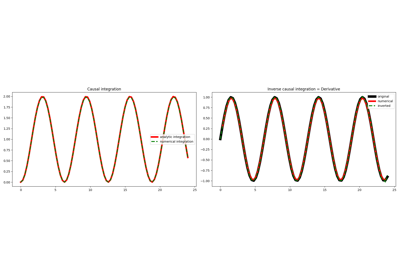Causal Integration

Causal Integration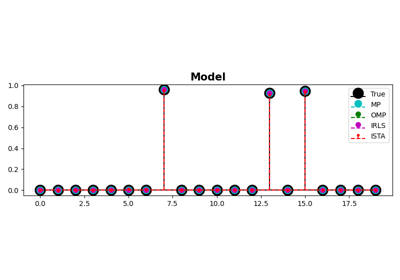MP, OMP, ISTA and FISTA

MP, OMP, ISTA and FISTA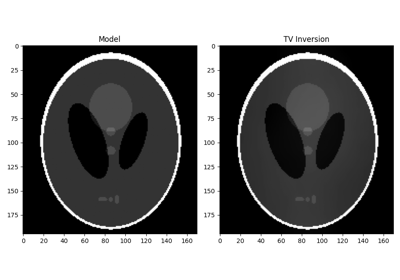Total Variation (TV) Regularization

Total Variation (TV) Regularization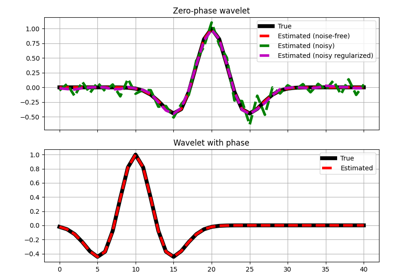Wavelet estimation

Wavelet estimation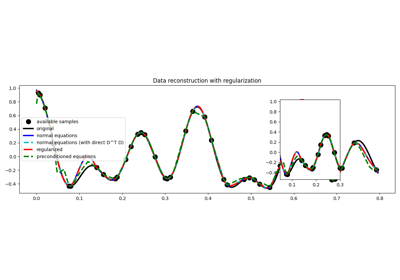03. Solvers

03. Solvers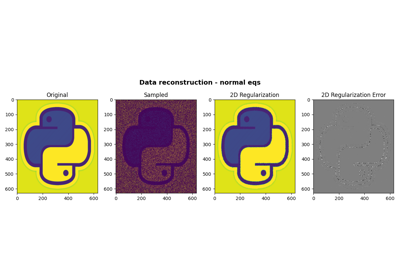06. 2D Interpolation

06. 2D Interpolation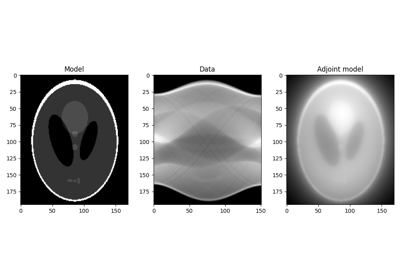16. CT Scan Imaging

16. CT Scan Imaging